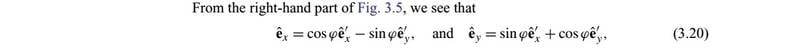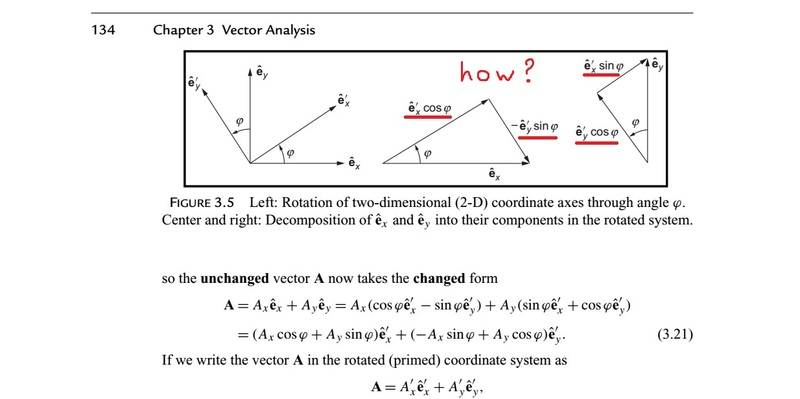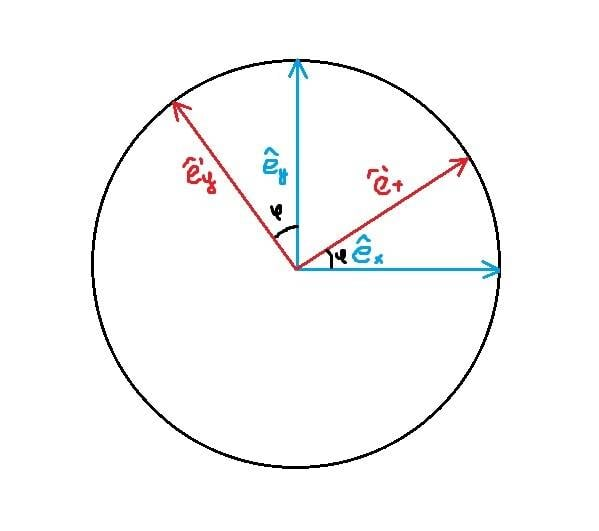# Coordinate transformation - Rotation

• I
• Quanta

#### QuantaHow author derives these old basis unit vectors in terms of new basis vectors ? Please don't explain in two words.

$\hat{e}_x = cos(\varphi)\hat{e}'_x - sin(\varphi)\hat{e}'_y$
$\hat{e}_y = sin(\varphi)\hat{e}'_x + cos(\varphi)\hat{e}'_y$​

It is just a matter of projecting the old basis on the new. Since the new basis is orthonormal, it holds that ##\vec e_i = (\vec e_i\cdot\vec e’_j)\vec e’_j##.

It is just a matter of projecting the old basis on the new. Since the new basis is orthonormal, it holds that ##\vec e_i = (\vec e_i\cdot\vec e’_j)\vec e’_j##.

We obtain:

$\vec{e}_i = (\vec{e}_i \cdot \vec{e}'_j)\vec{e}'_j$
$\vec{e}_i = | \vec{e}_i | | \vec{e}'_j |cos(\frac{\pi}{2}+\varphi) \vec{e}'_j$
$\vec{e}_i = -sin(\varphi)\vec{e}'_j$

Right ? But we didn't get transformation formula.

Last edited by a moderator:
No, you have not done it correctly. You cannot have different free indices on the different sides of the equation. Also note that repeated indices must be summed over.

No, you have not done it correctly. You cannot have different free indices on the different sides of the equation. Also note that repeated indices must be summed over.

$\vec{e}_1 = | \vec{e}_1 | | \vec{e}'_1 |cos(\varphi) \vec{e}'_1 + | \vec{e}_1 | | \vec{e}'_2 |cos(\frac{\pi}{2}+\varphi) \vec{e}'_2$
$\vec{e}_1 = cos(\varphi) \vec{e}'_1 -sin(\varphi)\vec{e}'_2$

$\vec{e}_2 = | \vec{e}_2 | | \vec{e}'_1 |cos(\frac{\pi}{2}-\varphi) \vec{e}'_1 + | \vec{e}_2 | | \vec{e}'_2 |cos(\varphi) \vec{e}'_2$
$\vec{e}_2 = sin(\varphi) \vec{e}'_1 +cos(\varphi)\vec{e}'_2$

Now am I right ?

But I want to understand in projection language (trigonometry) how author derives these formulas, but I didn't find explanation of unit vector basis transformation using trigonometry. I tried but didn't figured out how. Point me right direction.

The old basis vector is a unit vector that forms the hypothenuse of a right triangle who's other sides are its projections onto the new coordinate directions. The rest is just applying the definitions of sine and cosine in terms of the sides of a right triangle.

The other way around is to define a rotation as the transformation that relates two orthonormal bases. Looking at the two-dimensional case, you would have
$$\vec e_x = a \vec e'_x + b \vec e'_y \quad \mbox{and} \quad \vec e_y = c \vec e'_x + d \vec e'_y.$$
From here you can apply the orthonormality conditions of both systems in asserting that ##\vec e_x^2 = \vec e'^2_x = \vec e_y^2 = \vec e'^2_y = 1## and ##\vec e_x \cdot \vec e_y = \vec e'_x \cdot \vec e'_y = 0##. This will give you a set of relations among the coefficients ##a##, ##b##, ##c##, and ##d## (please derive these relations yourself). You should get three independent relations and since you have four parameters, you will be left with one free parameter (the angle of rotation). You will be able to make the interpretation of the angle of rotation by looking at the inner product between ##\vec e_x## and ##\vec e'_x##.

The old basis vector is a unit vector that forms the hypothenuse of a right triangle who's other sides are its projections onto the new coordinate directions. The rest is just applying the definitions of sine and cosine in terms of the sides of a right triangle.

What you said is evident from the picture, but how ? I drew it many times but cannot get sum of projections that is a old basis unit vector.

What you said is evident from the picture, but how ? I drew it many times but cannot get sum of projections that is a old basis unit vector.
I don't understand what you are trying to say here. It is evident from the picture but it is not evident?

I don't understand what you are trying to say here. It is evident from the picture but it is not evident?

How to project $\hat{e}_x$ on $\hat{e}'_x$ and $\hat{e}'_y$ ?Last edited by a moderator:
How to project $\hat{e}_x$ on $\hat{e}'_x$ and $\hat{e}'_y$ ?
Rotate your figure until $\hat{e}'_x$ is horizontal. Then the projections will be obvious.